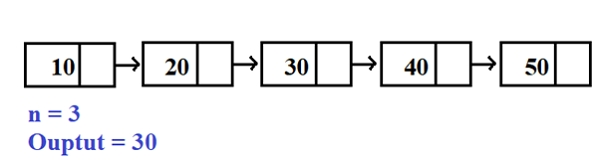# Program for n’th node from the end of a Linked List in C program

Given with n nodes the task is to print the nth node from the end of a linked list. The program must not change the order of nodes in a list instead it should only print the nth node from the last of a linked list.

## Example

Input -: 10 20 30 40 50 60
N=3
Output -: 40

In the above example, starting from first node the nodes till count-n nodes are traversed i.e 10,20 30,40, 50,60 and so the third node from the last is 40.Instead of traversing the entire list this efficient approach can be followed −

• Take a temporary pointer, let’s say, temp of type node
• Set this temp pointer to first node which is pointed by head pointer
• Set counter to the number of nodes in a list
• Move temp to temp → next till count-n
• Display temp → data

If we use this approach, than count will be 5 and program will iterate the loop till 5-3 i.e. 2, so starting from 10 on 0th location than to 20 on 1st location and 30 on 2nd location which is the result. So by this approach there is no need to traverse the entire list till end which will save space and memory.

## Algorithm

Start
Step 1 -> create structure of a node and temp, next and head as pointer to a structure node
struct node
int data
End
Step 2 -> declare function to insert a node in a list
void insert(int val)
struct node* newnode = (struct node*)malloc(sizeof(struct node))
newnode->data = val
End
Else
Loop While temp->next!=NULL
Set temp=temp->next
End
Set newnode->next=NULL
Set temp->next=newnode
End
Step 3 -> Declare a function to display list
void display()
Print no node
End
Else
Loop While temp!=NULL
Print temp->data
Set temp=temp->next
End
End
Step 4 -> declare a function to find nth node from last of a linked list
void last(int n)
declare int product=1, i
Loop For i=0 and i<count-n and i++
Set temp=temp->next
End
Print temp->data
Step 5 -> in main()
Create nodes using struct node* head = NULL
Declare variable n as nth to 3
Call function insert(10) to insert a node
Call display() to display the list
Call last(n) to find nth node from last of a list
Stop

## Example

Live Demo

#include<stdio.h>
#include<stdlib.h>
//structure of a node
struct node{
int data;
struct node *next;
int count=0;
//function for inserting nodes into a list
void insert(int val){
struct node* newnode = (struct node*)malloc(sizeof(struct node));
newnode->data = val;
newnode->next = NULL;
count++;
} else {
temp->next=newnode;
temp=temp->next;
count++;
}
}
//function for displaying a list
void display(){
printf("no node ");
else {
while(temp!=NULL) {
printf("%d ",temp->data);
temp=temp->next;
}
}
}
//function for finding 3rd node from the last of a linked list
void last(int n){
int i;
for(i=0;i<count-n;i++){
temp=temp->next;
}
printf("%drd node from the end of linked list is : %d" ,n,temp->data);
}
int main(){
//creating list
int n=3;
//inserting elements into a list
insert(1);
insert(2);
insert(3);
insert(4);
insert(5);
insert(6);
//displaying the list
display();
//calling function for finding nth element in a list from last
last(n);
return 0;
}

## Output

linked list is : 1 2 3 4 5 6
3rd node from the end of linked list is : 4

Updated on: 04-Jul-2020

976 Views